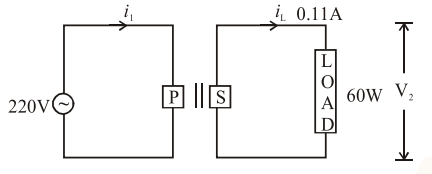# For the given circuit, comment on the type of transformer used :

Question:

For the given circuit, comment on the type of transformer used :1. Auxilliary transformer

2. Auto transformer

3. Step-up transformer

4. Step down transformer

Correct Option:

Solution:

$\mathrm{V}_{\mathrm{S}}=\frac{\mathrm{P}}{\mathrm{i}}=\frac{60}{0.11}=545.45$

$\mathrm{V}_{\mathrm{P}}=220$

$\mathrm{~V}_{\mathrm{S}}>\mathrm{V}_{\mathrm{P}}$

$\Rightarrow$ Step up transformer